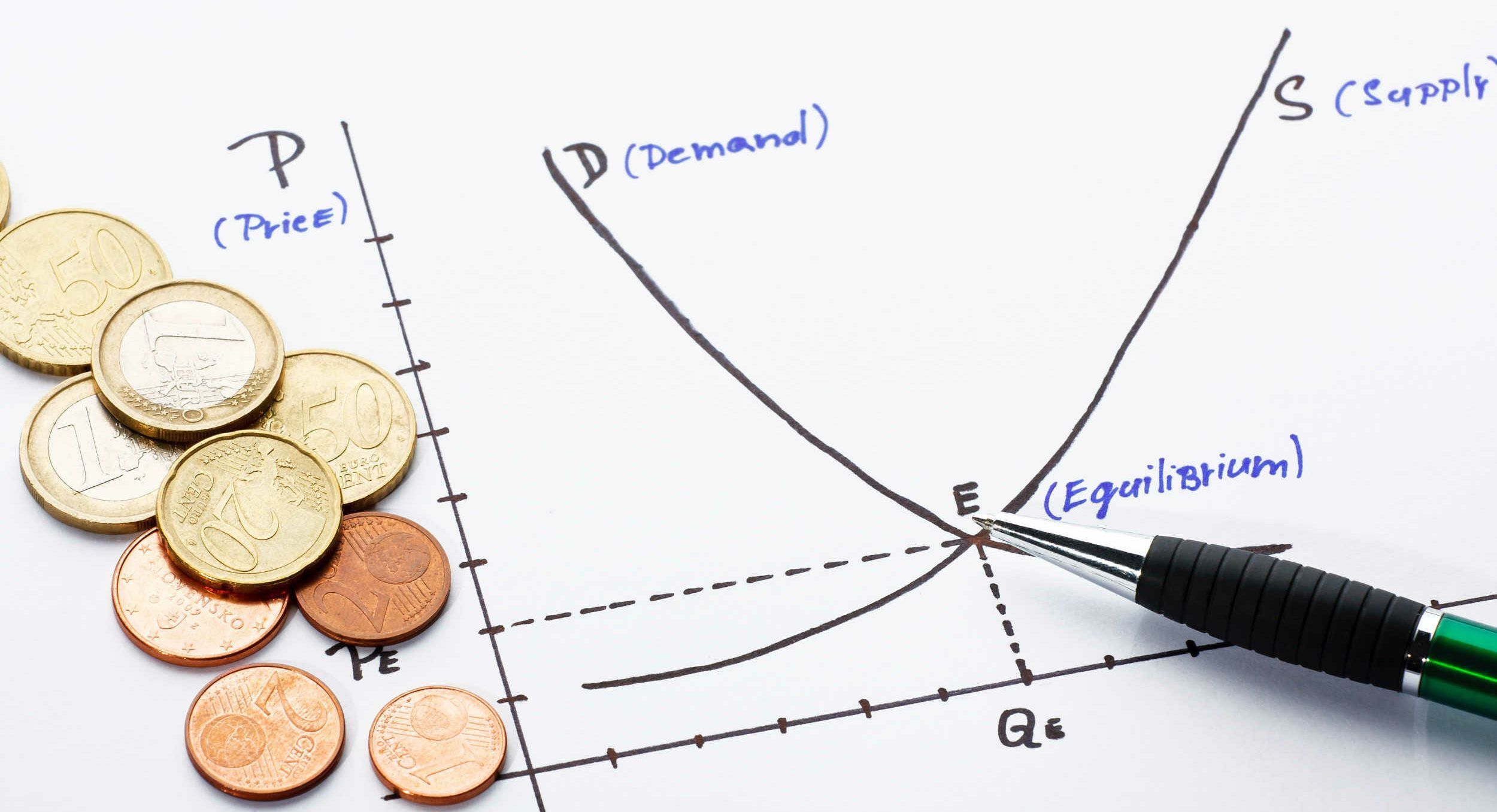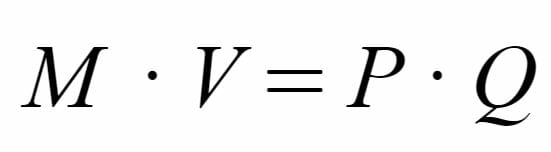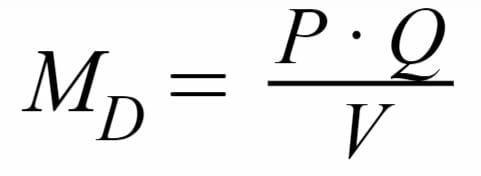# Quantity Theory of Money

Understanding the relationship between money supply and price levels

## What is the Quantity Theory of Money?

The Quantity Theory of Money refers to the idea that the quantity of money available (money supply) grows at the same rate as price levels do in the long run. When interest rates fall or taxes decrease and the access to money becomes less restricted, consumers become less sensitive to price changes and, thus, will have a higher propensity to consume. As a result, the aggregate demand curve will shift right, thus shifting up the equilibrium price level.### Exchange Equation

To better understand the Quantity Theory of Money, we can use the Exchange Equation. The equation enables economists to model the relationship between money supply and price levels. The exchange equation is:Where:

M – refers to the money supply

V – refers to the Velocity of Money, which measures how much a single dollar of money supply spend contributes to GDP

P – refers to the prevailing price level

Q – refers to the quantity of goods and services produced in the economy

Holding Q and V constant, we can see that increases in the money supply will cause price levels to increase, thus causing inflation. The assumption that Q and V are constant holds in the long run as these factors cannot be influenced by changes in the economy’s money supply.

The theory provides a quick overview of monetarist theory, which states that changes in the current money supply cause fluctuations in overall economic output; excessive growth in money supply causes hikes in inflation.

### Demand for Money

The Exchange Equation can also be remodeled into the Demand for Money equation as follows:Where:

Md – Refers to the demand for money

P – refers to the price level in the economy

Q – refers to the quantity of goods and services offered in the economy

V – refers to the Velocity of Money

In the formula, the numerator term (P x Q ) refers to the nominal GDP of a country. Moreover, the equation provides another take on the monetarist theory as it relates GDP to the demand for money (contrary to Keynesian economists, who believe that interest rates drive inflation).

### More Resources

CFI offers the Financial Modeling & Valuation Analyst (FMVA)™ certification program for those looking to take their careers to the next level. To learn more about related topics, check out the following CFI resources:

• Inflation
• Inelastic Demand
• Price Elasticity
• Supply and Demand

### Financial Analyst Certification

Become a certified Financial Modeling and Valuation Analyst (FMVA)® by completing CFI’s online financial modeling classes!